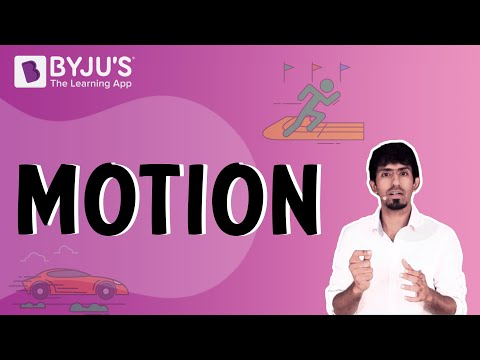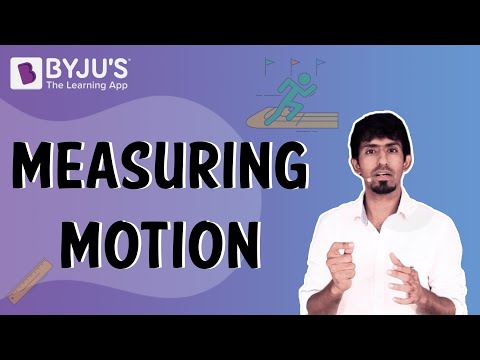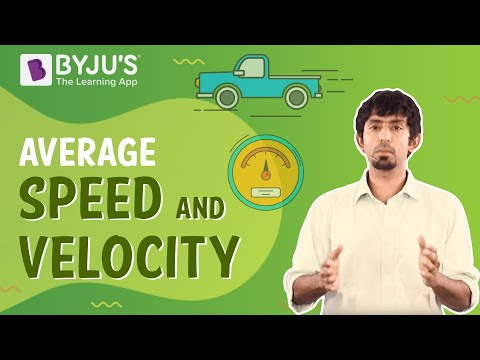# Motion and Time Class 7 Science Notes - Chapter 13

According to the CBSE Syllabus 2023-24, this chapter has been renumbered as Chapter 9.

## An Introduction to Motion

### Distance

• Distance is the total path covered by the object in the given interval of time.
• Displacement is the shortest path covered by the moving object in the given interval of time.
• Distance=Speed × Time.
• Unit of distance is generally measured in units of length like metres, kilometres etc.

### Introduction to Motion

• An object is said to be in motion if it changes its position with respect to time. For example, A car moving on a road.
• An object is said to be at rest if the object does not change its position with time. For example, A person standing on the ground.To know more about Motion, visit here.

## Basics of Standard Units

### Units and their standardization

• The standard unit of distance is in metres.
• The standard unit of time is in seconds (s).
• The standard unit of speed is in metres per second (m/s).

To know more about Standard Units of Measurement, visit here.

## Basics of Motion and Its Types

### Types of motion

Types of motion are generally divided into 3. They are:

1. Rectilinear/translatory motion: When a body is moving in a straight line without changing its direction, then the body is said to possess translatory/rectilinear motion.
For example,  A car moving on a straight road.

2. Circular motion: When a body moves in the shape of a circle about a fixed point and a fixed radius, then the body is said to be in a circular motion.
For example, the motion of planets around the sun.

3. Period / Oscillatory motion: When the motion of a body repeats after fixed intervals of time, then the body is said to be in the periodic/oscillatory motion.
For example, the To and fro motion of a simple pendulum.

To know more about the Type of Motion, visit here.

### Periodic or Oscillatory Motion

• Periodic or oscillatory motion is the motion in which a body repeats its motion after fixed intervals of time.
• For example, the To and fro motion of a  simple pendulum, the Motion of a car in a  circular path, Motion of planets around the sun.

To know more about Oscillatory Motion, visit here.

### Oscillations of a Simple Pendulum

• When the bob of a simple pendulum moves from its mean position B to A and back to B again, then from B to C and back to B again, the pendulum is said to complete 1 oscillation.
• In the case of the simple pendulum, the time period is the total time taken by the pendulum to complete one oscillation.

## Time and Speed

### Speed (Average Speed)

• Speed is the total distance travelled by the object in a given interval of time.
• Speed = Distance travelled/time taken.
• The unit of speed is generally measured in metres per second(m/s), kilometres per hour(km/h).

#### For more information on Average Speed and Velocity, watch the below video### Uniform and Non-Uniform Motion

1. Uniform motion: When an object moving along a straight line moves with a constant speed, then the object is said to be in uniform motion.
For example, A car moving in a straight line with a constant speed.

2. Non-uniform motion: When an object moving along a straight line changes its speed with respect to time, then the object is said to be in uniform motion.
For example, The motion of a train.

#### For more information on Uniform and Non-Uniform Motion, watch the below videoTo know more about Uniform and Non-Uniform Motion, visit here.

### Measurement of Time

• The unit of time is generally measured in seconds, minutes, and hours.
• The time period is the total time taken by an object to complete one oscillation.

### Units of Time

The unit of time is generally measured in seconds, minutes, and hours.

### Units of Speed

• Speed = Distance travelled in the total time interval.
• The unit of speed is generally measured in metres per second (m/s) and km/h( kilometre per hour).

### Speedometer and Odometer

• The speedometer records the speed of the vehicle directly in kilometres per hour (km/h).
• An odometer measures the distance moved by the vehicle directly in kilometres(km).

## Visualizing Motion

### Distance-time graph

• A distance-time graph is a graph plotted between the distance( in the y-axis) and time ( in the x-axis).
• The slope of a distance-time graph gives the speed of an object.

## Frequently Asked Questions on CBSE Class 7 Science Notes Chapter 13 Motion and Time

Q1

### What is rectilinear motion?

It is a type of linear motion in which the direction of the velocity remains constant, and the path is a straight line.

Q2

### What is the average speed?

Average speed is calculated by dividing the total distance that something has travelled by the total amount of time it took it to travel that distance.

Q3

### What are the uses of uniform motion?

If an object is travelling with uniform motion, it is moving in a straight line at a constant speed.